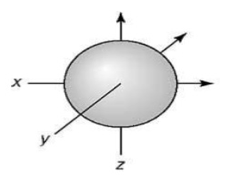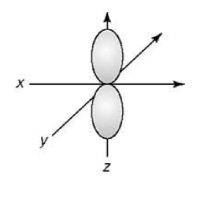Ch.8 - Periodic Properties of the ElementsWorksheetSee all chapters
 Ch.1 - Intro to General Chemistry 2hrs & 53mins 0% complete Worksheet Ch.2 - Atoms & Elements 2hrs & 49mins 0% complete Worksheet Ch.3 - Chemical Reactions 3hrs & 25mins 0% complete Worksheet BONUS: Lab Techniques and Procedures 1hr & 38mins 0% complete Worksheet BONUS: Mathematical Operations and Functions 47mins 0% complete Worksheet Ch.4 - Chemical Quantities & Aqueous Reactions 3hrs & 55mins 0% complete Worksheet Ch.5 - Gases 3hrs & 47mins 0% complete Worksheet Ch.6 - Thermochemistry 2hrs & 28mins 0% complete Worksheet Ch.7 - Quantum Mechanics 2hrs & 35mins 0% complete Worksheet Ch.8 - Periodic Properties of the Elements 1hr & 57mins 0% complete Worksheet Ch.9 - Bonding & Molecular Structure 2hrs & 5mins 0% complete Worksheet Ch.10 - Molecular Shapes & Valence Bond Theory 1hr & 31mins 0% complete Worksheet Ch.11 - Liquids, Solids & Intermolecular Forces 3hrs & 40mins 0% complete Worksheet Ch.12 - Solutions 2hrs & 17mins 0% complete Worksheet Ch.13 - Chemical Kinetics 2hrs & 22mins 0% complete Worksheet Ch.14 - Chemical Equilibrium 2hrs & 26mins 0% complete Worksheet Ch.15 - Acid and Base Equilibrium 4hrs & 42mins 0% complete Worksheet Ch.16 - Aqueous Equilibrium 3hrs & 48mins 0% complete Worksheet Ch. 17 - Chemical Thermodynamics 1hr & 44mins 0% complete Worksheet Ch.18 - Electrochemistry 2hrs & 58mins 0% complete Worksheet Ch.19 - Nuclear Chemistry 1hr & 33mins 0% complete Worksheet Ch.20 - Organic Chemistry 3hrs 0% complete Worksheet Ch.22 - Chemistry of the Nonmetals 2hrs & 1min 0% complete Worksheet Ch.23 - Transition Metals and Coordination Compounds 1hr & 54mins 0% complete Worksheet

Each atomic orbital has designated shapes that are determined by the angular momentum quantum number (l)

###### Atomic Orbital Shape

Concept #1: Quantum Numbers and Atomic Orbital Shape

Transcript

In this new video, we're going to continue with atomic shape. Remember, we said that the second quantum number, which is known as our angular momentum quantum number, tells us the shape of a particular orbital. Each one has a specific shape associated with it. Remember we can also call this our azimuthal or subsidiary quantum number. All three terms mean the same thing.
Now, we're going to say it uses the variable l and the formula n minus one. We're going to say each sublevel has its own l value associated with it. If we know the sublevel or sub-shell letter, then we know what the l value is. S is zero, p is one, d is two, f is three, g is four, h is five.Example #1: Based on the following atomic orbital shape, which of the following set of quantum numbers is correct:

a) n = 8, l = 1, ml = 1/2

b) n = 8, l = 2, ml = -2

c) n = 8, l = 0, ml =  1

d) n = 8, l = 0, ml = 0Practice: Based on the following atomic orbital shape, which of the following set of quantum numbers is correct:

a) n = 2, l = 1, ml = +1, ms = -1

b) n = 4, l = 1, ml = -2, ms = +1/2

c) n = 3, l = 1, ml = -1, ms = 0

d) n = 2, l = 1, ml = +1, ms = -1/2